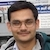# NAEST 2015 Screening Test: Conical PendulumBy

Pass a thread through plastic body of a used pen. Tie two unequal masses m and M on the two sides of the thread. Hold the plastic body in the vertical position in your hand with the heavier mass M hanging and the lighter mass m resting at the top of the plastic body. Give motion to the masses by rotating your hand a little bit so that the upper mass is set in a nearly circular motion. As soon as it acquires sufficient speed it will pull the hanging body up. If you speed up the rotating body the heavier hanging mass can move right up to the plastic body. You can adjust the speed of the rotating body by manipulating the force provided by your hand. By properly adjusting this force, you can keep the hanging body fixed at a desired height.

Question 1: The balance shown in video measure mass in grams. Calculate the tension in the string when the hanging mass is stationary and rotating mass (eraser) rotates at constant speed?

Question 2: The hanging mass starts rising up when the eraser is rotated faster. Explain?

Question 3: When hanging mass is pulled down by hand, the eraser starts rotating faster. Why?

Answer: The tension in the string is 0.284 N = 0.29 N (upwards). The mass of the bob (as shown in video) is 29 g. When hanging mass (bob) is stationary, its weight is balanced by the tension in the string. Hence, tension \begin{align} T =mg=0.029\times 9.8\approx 0.29\, \text{N}. \end{align}

Let us analyse the motion of eraser (rotating mass). It rotates in almost a horizontal circle. The tension in the string provides centripetal force ($m \omega^2 r$) for the rotational motion, where m is mass of the eraser, $\omega$ is angular speed, and r is distance from the axis of rotation. The centripetal force (string tension) increases when $\omega$ is increased. Thus, tension is greater than the weight of hanging mass and hence it starts rising upward.

The angular speed increases when the thread is pulled down. This can be explained by the conservation of angular momentum.